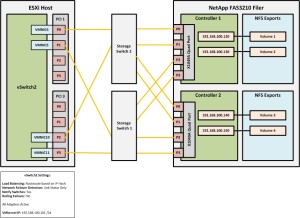# Troubleshooting IP-Hash load balancing on a NFS storage network.

When configuring a NFS storage network at one of our customers some time ago, I noticed that the ESXi host wasn’t utilizing all NICs assigned to the NIC team for the VMkernel traffic. After some research, I have found this article written by Frank Denneman a while ago and this VMware KB document. According to the blog post and the article mentioned above, this issue may occur if the calculated hash returns the same result based on the source IP and both destination IP’s. Before we jump in to troubleshooting, let’s take a look at what is exactly going wrong.

# Setup

The setup consisted of 4 Dell R710 ESXi hosts connected through 2 stacked Cisco 2960 switches to a NetApp FAS3210 filer. Four NICs per server have been dedicated to NFS storage and cabled in a redundant configuration (2 per switch in EtherChannel). See the drawing for more details.# Solution

To see what is going wrong, we need to calculate the IP-Hash manually. The formula is:

Source IP XOR Destination IP = x MOD y = z where:

Source IP = VMkernel IP address in Hexadecimal
Destination IP = IP address of the NFS filer in Hexadecimal
x = Exclusive OR operation output
y = Number of physical NICs
z = Modulo operation output

First, let’s calculate the IP-Hash value of the IP addresses in the current setup. To do this, we need to convert the IP addresses from decimal to hexadecimal. I used the BitCricket IP Calculator to do the conversion.Next, calculate the IP-Hash with the formula specified earlier and take a look at the outcome. You can use Windows Calculator to do this, just set the view to Programmer and make sure it is set to Hex and Qword.

1.    C0A86465 XOR C0A86478 = 1D MOD 4 = 1
2.    C0A86465 XOR C0A86482 = E7 MOD 4 = 3
3.    C0A86465 XOR C0A8648C = E9 MOD 4 = 1
4.    C0A86465 XOR C0A86496 = F3 MOD 4 = 3

As you can see, the values are not unique. That’s what causes the problem. The IP-Hash calculation only returns 2 different values instead of 4. To correct this, we need to reconfigure the destination IP addresses (on the NFS Filer) so that every IP-Hash calculations return a unique value. The IP addresses have been reconfigured as follow:Let’s have a look at the IP-Hash calculations now.

1.    C0A86465 XOR C0A8646F = A MOD 4 = 2
2.    C0A86465 XOR C0A86470 = 15 MOD 4 = 1
3.    C0A86465 XOR C0A86471 = 14 MOD 4 = 0
4.    C0A86465 XOR C0A86472 = 17 MOD 4 = 3

As you can see, the IP-Hash calculation now returns unique values in all four cases. This will now allow utilization of all four NICs from the ESXi host to the NFS Filer.

Cheers!

– Marek.Z